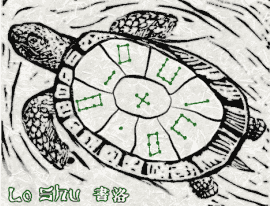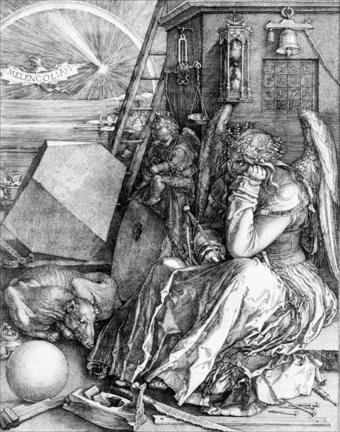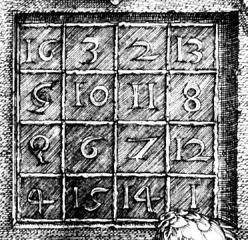# Magic Squares, Part 1, Low Order

With origins in centuries old recreational mathematics, magic squares demonstrate MATLAB array operations.

### Contents

#### Magic Squares

An n -by- n magic square is an array containing the integers from 1 to $n^2$, arranged so that each of the rows, each of the columns, and the two principal diagonals have the same sum. For each $n > 3$, there are many different magic squares of order n. The MATLAB function magic(n) generates one particular one. There is no magic square of order $n = 2$. The statement
A = magic(2)

produces
A =

     1     3
4     2
The column sums are the same, but the row and diagonal sums are not, so this is not a magic square.

#### Lo Shu(Illustration thanks to Byerly Wiser Cline.) Magic squares predate recorded history. An ancient Chinese legend tells of a turtle emerging from the Lo river during a flood. The turtle's shell showed a very unusual pattern -- a 3-by-3 grid containing various numbers of spots. Of course, we do not have any eye-witness accounts, so we can only imagine what the turtle looked like. Each of the three rows, the three columns, and the two diagonals contain a total of 15 spots. References to Lo Shu and the Lo Shu numerical pattern occur throughout Chinese history. Today, it is the mathematical basis for Feng Shui, the philosophy of balance and harmony in our surroundings and lives. MATLAB can generate Lo Shu with
A = magic(3)

This produces
A =
8     1     6
3     5     7
4     9     2

The command
sum(A)

sums the elements in each column to produce
     15    15    15
The command
sum(A')'

transposes the matrix, sums the columns of the transpose, and then transposes the results to produce the row sums
     15
15
15
The command
    sum(diag(A))
sums the main diagonal of A, which runs from upper left to lower right, to produce 15. The opposite diagonal, which runs from upper right to lower left, is less important in linear algebra, so finding its sum is a little trickier. One way to do it makes use of the function that "flips" a matrix "upside-down."
     sum(diag(flipud(A)))
produces 15. This verifies that A has equal row, column, and diagonal sums and so is a magic square. Why is the magic sum equal to 15? The command sum(1:9) tells us that the sum of the integers from 1 to 9 is 45. If these integers are allocated to 3 columns with equal sums, that sum must be sum(1:9)/3 which is 15. There are eight possible ways to orient a photo in a slide show. Similarly, there are eight possible ways to display the magic square of order 3. The statements
   for k = 0:3
rot90(A,k)
rot90(A',k)
end
produce the eight displays
    8     1     6        8     3     4
3     5     7        1     5     9
4     9     2        6     7     2
    6     7     2        4     9     2
1     5     9        3     5     7
8     3     4        8     1     6
    2     9     4        2     7     6
7     5     3        9     5     1
6     1     8        4     3     8
    4     3     8        6     1     8
9     5     1        7     5     3
2     7     6        2     9     4
The 5 is always in the center, the other odd numbers are always in the centers of the edges, and the even numbers are always in the corners.

#### MelancholiaMelancholia I is a famous Renaissance engraving by the German artist and amateur mathematician Albrecht Dürer. It shows many mathematical objects, including a sphere, a truncated rhombohedron, and, in the upper right hand corner, a magic square of order 4. Issue these MATLAB commands
   load durer
whos
You will see
   X           648x509        2638656  double array
caption       2x28             112  char array
map         128x3             3072  double array
The elements of the array X are indices into the gray-scale color map named map. The image is displayed with
   image(X)
colormap(map)
axis image
Click the magnifying glass with a "+" in the toolbar and use the mouse to zoom in on the magic square in the upper right-hand corner. The scanning resolution becomes evident as you zoom in. To display the higher resolution scan of the area around the magic square use the commands
   load detail
image(X)
colormap(map)
axis image
You will seeThe command
   A = magic(4)
produces a 4-by-4 magic square.
   A =
16     2     3    13
5    11    10     8
9     7     6    12
4    14    15     1
The commands
   sum(A), sum(A'), sum(diag(A)), sum(diag(flipud(A)))
yield enough 34's to verify that A is indeed a magic square. The 4-by-4 magic square generated by MATLAB is not the same as Dürer's magic square. We need to interchange the second and third columns.
   A = A(:,[1 3 2 4])
changes A to
   A =
16     3     2    13
5    10    11     8
9     6     7    12
4    15    14     1
Interchanging columns does not change the column sums or the row sums. It usually changes the diagonal sums, but in this case both diagonal sums are still 34. So now our magic square matches the one in Dürer's etching. Dürer probably chose this particular 4-by-4 square because the date he did the work, 1514, occurs in the middle of the bottom row. There are 880 different magic squares of order 4. To see some of them, run this:
A = magic(4);
while 1
clc
A = A(randperm(4),randperm(4))
pause(.5)
end

The program durerperm available from Experiments with MATLAB interchanges rows and columns in the image produced from detail by interchanging groups of rows and columns in the array X. This is not especially important or useful, but it provides a curious illusion.

Published with MATLAB® R2012b

|